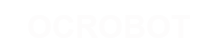#### Site Tools

en:reference:language:boolean

# Boolean Operators

These can be used inside the condition of an if statement.

## && (logical and)

True only if both operands are true, e.g.

```if (digitalRead(2) == HIGH  && digitalRead(3) == HIGH) { // read two switches
// ...
} ```

is true only if both inputs are high.

## || (logical or)

True if either operand is true, e.g.

```if (x > 0 || y > 0) {
// ...
} ```

is true if either x or y is greater than 0.

## ! (not)

True if the operand is false, e.g.

```if (!x) {
// ...
} ```

is true if x is false (i.e. if x equals 0).

## Warning

Make sure you don't mistake the boolean AND operator, && (double ampersand) for the bitwise AND operator & (single ampersand). They are entirely different beasts.

Similarly, do not confuse the boolean || (double pipe) operator with the bitwise OR operator | (single pipe).

The bitwise not ~ (tilde) looks much different than the boolean not ! (exclamation point or “bang” as the programmers say) but you still have to be sure which one you want where.

## Examples

`if (a >= 10 && a <= 20){}   // true if a is between 10 and 20`